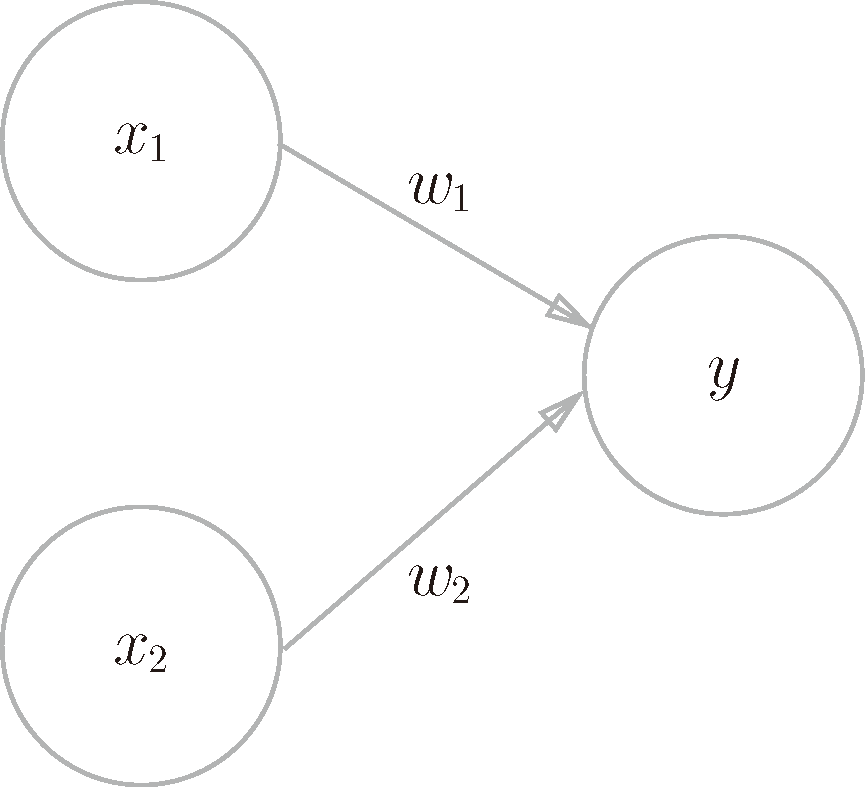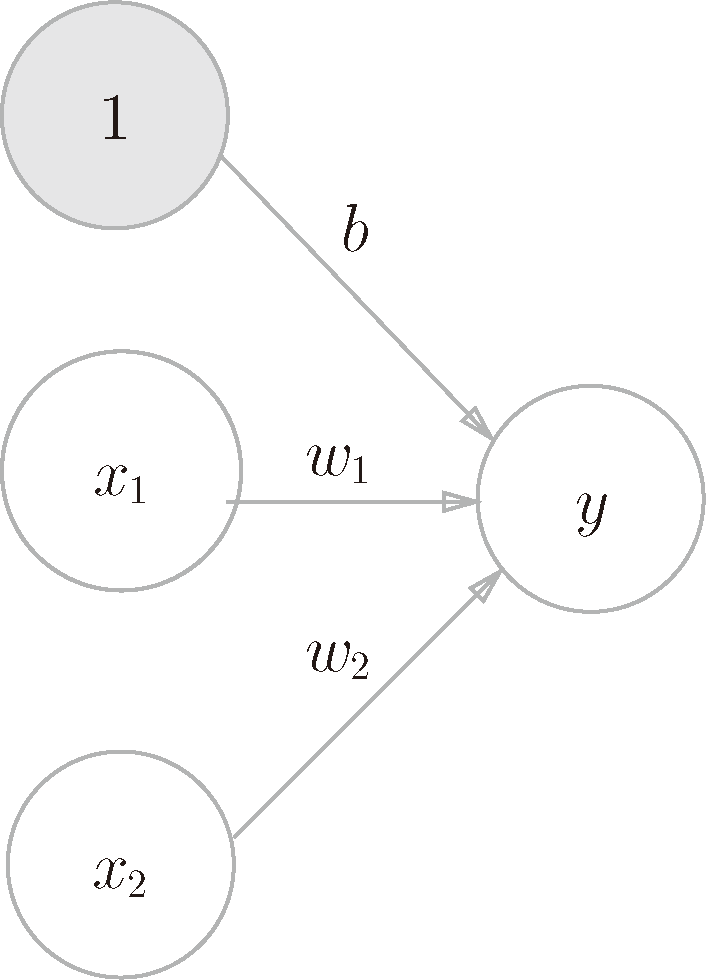# 深度学习入门：基于 Python 的理论与实现 (3)：神经网络 3.1.2(复习感知机)$y=\begin{cases}0&(b+w_1x_1+w_2x_2\leqslant0)\1&(b+w_1x_1+w_2x_2>0)\end{cases}\quad\quad\quad\quad\quad(3.1)$

$b$ 是被称为偏置的参数，用于控制神经元被激活的容易程度；而 $w_1$$w_2$ 是表示各个信号的权重的参数，用于控制各个信号的重要性。

$y=h(b+w_1x_1+w_2x_2)\quad\quad\quad\quad\quad(3.2)$$h(x)=\begin{cases}0&(x\leqslant0)\1&(x>0)\end{cases}\quad\quad\quad\quad\quad(3.3)$